APPLIED INDUSTRIAL CONTROL SOLUTIONS LLCPAPER No. 12

Winder/Unwinder Scheduling Equations

2003 IEEE IAS Annual Meeting

Brian Thomas Boulter
Applied Industrial Control Solutions LLC
4597 E Sprague Rd.
Independence, OH, USA 44131

Abstract- Non-recursive analytic equations describing the relationship between translational speed, translational acceleration rates, material thickness, and roll-diameter as a function of time are presented. These equations can be used to estimate winding & unwinding times for scheduling product in winder and unwinder entry and exit sections of web handling and strip processing lines. They can also be used to minimize jog time by estimating the optimal diameter at which to begin line deceleration at core in unwinding applications, and full roll in winding applications.

I. Introduction

A. Nomenclature

 TH Strip/web thickness [m] W Roll width [m] wR Roll rotational speed [rad/sec] wM Motor rotational speed [rad/sec] V Roll surface speed [m/sec] ACC Roll surface speed acceleration [m/sec^2] DEC Roll surface speed deceleration [m/sec^2] Ri Roll radius [m] REC Empty core radius [m] RFR Full roll radius [m] tM Motor torque [Nm] GRM Motor gear ratio D 2 x Ri , Roll diameter [m] Dt tFINAL – 0, or Delta time [sec]

Roll diameter configuration definitions: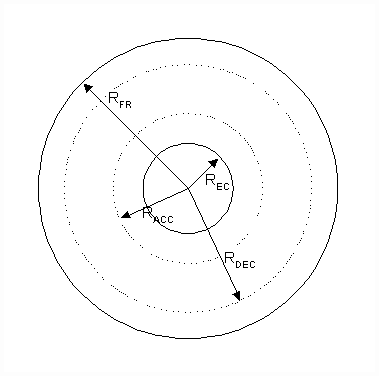Fig. 1 Winding Applications Roll Configuration

In winding applications the diameter at which Line acceleration ends is defined as RACC, and occurs near core. The diameter at which Line deceleration starts is defined as RDEC., and occurs near full roll.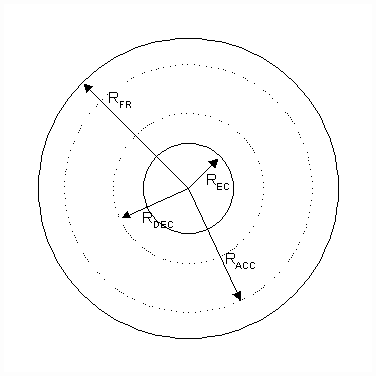Fig. 2 Unwinding Applications Roll Configuration

In unwinding applications the diameter at which Line acceleration ends is defined as RACC, and occurs near full roll. The diameter at which Line deceleration starts is defined as RDEC., and occurs near core.

Paper Overview

The time domain equations that describe winding and unwinding of rolls are recursive and given below.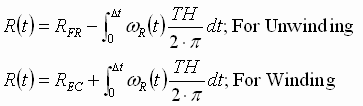; (1)

Where, assuming a perfect transmission system, and no clutch or roll winding slippage, the motor and roll rotational speeds are defined as: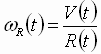; (2)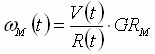; (3)

The author has derived a simple set of exact analytic equations that solve the above recursive equations and provide the control engineer an equation set which can be used for calculating approximate winding and unwinding times and estimating the roll diameter at any point in time during the build-up/wind-down of the roll. This is accomplished without the need to solve (1) and (2) with a numerical integration based equation solver.

The paper is divided into four sections. The first section is composed of this introduction and the paper nomenclature, the second section defines the equation set, the third section provides some useful examples. Observations and conclusions are presented in the fourth section

II. The Equation Set

The tedious derivations of the following equations are not presented in this paper, and are left up to the interested reader as an exercise.

A. Diameter during accelerating line speed

It can be shown that for a given web/strip thickness, a given starting roll radius, and a given accelerating line-speed the following equations (4a,b) yield R(t), the roll radius as a function of time, and RACC, the roll radius after Dt [sec] of acceleration. Note that the units must be consistent. If the units described in the nomenclature are used they will be consistent.

 Winding Unwinding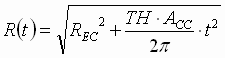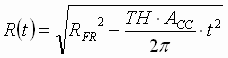(4a)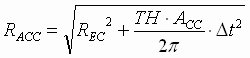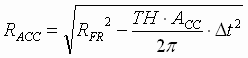(4b)

The time for acceleration can be computed with Eq. (5), assuming consistent units.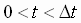;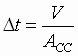(5)

B. Calculating the "Start deceleration" diameter

It can be shown that the diameter at which to begin deceleration is calculated as a function of the line speed from which the deceleration begins, Eq. (6).

 Winding Unwinding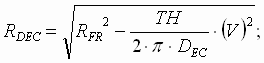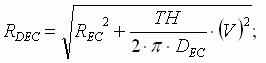(6)

Where RDEC is the roll radius at which to begin deceleration, and RFR and REC are the target ending roll radii.

C. Diameter during constant line speed

It can be shown that Eq’s (7a,b) yield R(t), the roll radius as a function of time, and RDEC, the roll radius after Dt [sec] of constant translational line speed run time.

 Winding Unwinding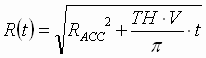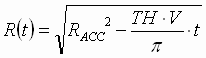(7a)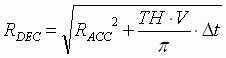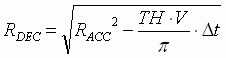(7b)

Where RDEC is the radius of the roll when deceleration must take place, found with Eq. (7b). RACC is the radius of the roll at the end of line acceleration, found with Eq. (4b).

The constant translational speed winding/unwinding time can be calculated as a function of known radii, calculated with Eq’s (4b) and (6):;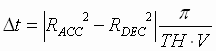(8)

Note that RDEC obtained with Eq’s (6) and (7b) is consistent.

D. Diameter during decelerating line speed

It can be shown that the equations describing the change in diameter during deceleration (9a,b) are:

 Winding Unwinding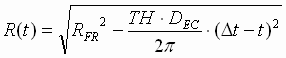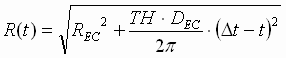(9a)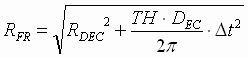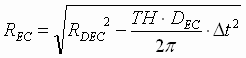(9b)

The time for deceleration can be computed with (10), assuming consistent units.;(10)

E. Rotational velocity during acceleration

It can be shown that the rotational speed of the roll during line acceleration from a stalled condition can be calculated as a function of time (11).

 Winding [rad/sec] Unwinding [rad/sec]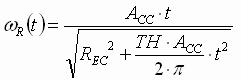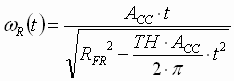;(11)

In units of [rpm]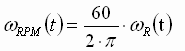Where REC and RFR are the radius of the roll at t=0.

F. Rotational velocity during line run

It can be shown that the rotational speed of the roll during the constant translational line speed run time can be calculated as a function of time (12).

 Winding [rad/sec] Unwinding [rad/sec]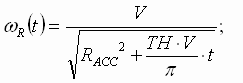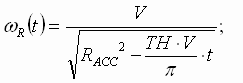(12);G. Rotational velocity during deceleration

It can be shown that the rotational speed of the roll during line deceleration, Eq. (13), is:

 Winding [rad/sec] Unwinding [rad/sec]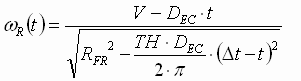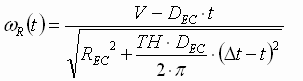(13);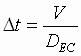H. Maximum Rotational Velocity

The maximum rotational velocity will occur at core. For winding applications this will occur at the end of the line acceleration. For unwinding applications this will occur at the beginning of line deceleration. Eq. (14) computes these two values using Eq’s (3,4b,6).

 Winding [rad/sec] Unwinding [rad/sec]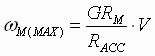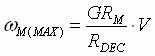; (14)

III. Three Examples

The following examples are for a system with the following roll and line-speed profiles. The slow acceleration and deceleration rates are not typical, and are chosen for the purpose of demonstration only:

 Line speed 1000 [m/min] GR 5 ACC 5 [m/min/sec] DEC 5 [m/min/sec] TH 0.0005 [m] REC 0.1 [m] RFR 1.5 [m]

Example 1. Roll buildup from empty core to full roll

The following example demonstrates how to combine the above equations to calculate the roll time for a roll buildup from core to full roll. Graphs of the roll build-up can be computed using the described equations.

Step 1: Calculate TACC: Eq. (5); TACC = 200 [sec]

Step 2: Calculate RACC: Eq. (4b); RACC = 0.525 [m]

Step 3: Calculate RDEC: Eq. (6); RDEC = 1.406 [m]

Step 4: Calculate TRUN: Eq. (8); TRUN = 644 [sec]

Step 5: Calculate TDEC: Eq. (10); TDEC = 200 [sec]

Step 6: Calculate run time: TACC+ TRUN+TDEC = 1044 [sec]

A graph (Fig. 3) of motor speed as a function of time for winding applications can be created using Eq’s (11,12,13).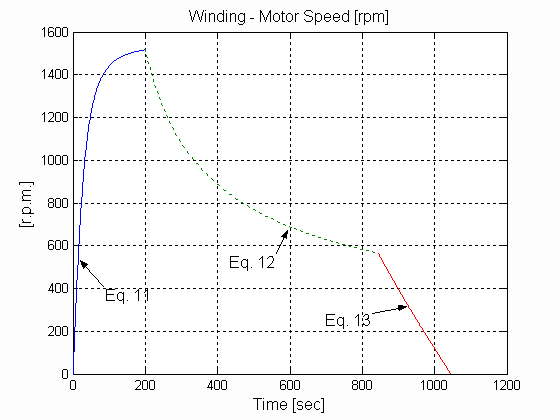Fig. 3. Winding Motor Speed

A graph (Fig. 4) of diameter build-up with time can also be created using Eq’s (4a, 7a, 9a).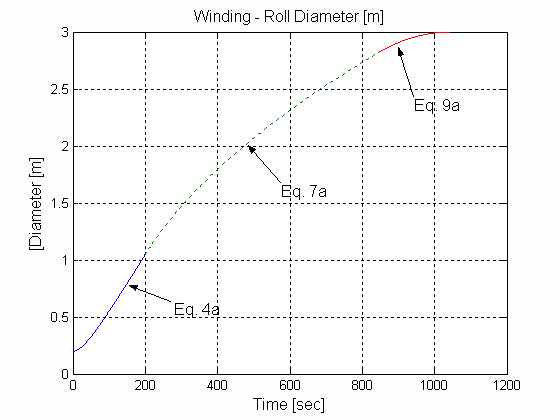Fig. 4. Winding Roll Diameter

Example 2. Roll unwind from full roll to empty core

The following example demonstrates computations for a roll wind-down from full roll to core. Graphs of the roll unwinding can be computed using the described equations.

Step 1: Calculate TACC: Eq. (5); TACC = 200 [sec]

Step 2: Calculate RACC: Eq. (4b); RACC = 1.406 [m]

Step 3: Calculate RDEC: Eq. (6); RDEC = 0.525 [m]

Step 4: Calculate TRUN: Eq. (8); TRUN = 644 [sec]

Step 5: Calculate TDEC: Eq. (10); TDEC = 200 [sec]

Step 6: Calculate run time: TACC+ TRUN+TDEC = 1044 [sec]

A graph (Fig. 5) of motor speed as a function of time for unwinding applications can be created using Eq’s (11,12,13).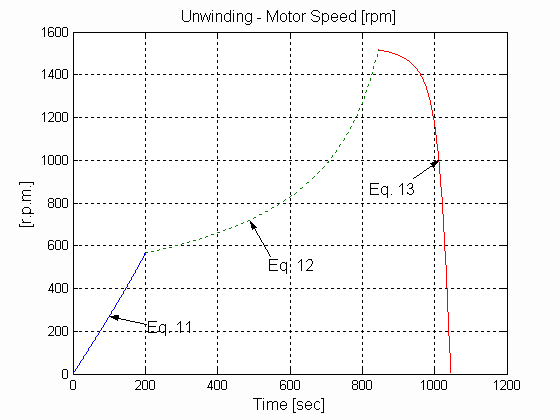Fig. 5. Unwinding Motor Speed

A graph of diameter wind-down with time can also be created using Eq’s (4a, 7a, 9a), as shown in Fig. 6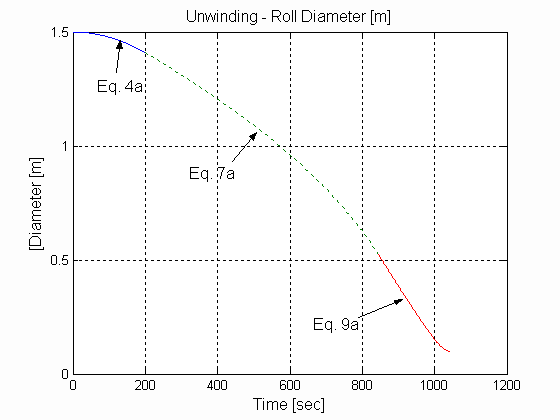Fig. 6. Unwinding Roll Diameter

Example 3. Sizing a Winder/Unwinder motor.

The described equations can also be useful when prescribing a winder/unwinder motor.

In applications where the ratio of RFR to REC is greater than the ratio of the fully-weakened-field motor speed to the motor base-speed, the motor is sized to provide enough torque to deliver application maximum tension at RFR. And the motor base speed and/or gearing are adjusted to meet the speed requirement at core. To overcome the need for increasing the motor base speed and/or lowering the gearing, and consequently increasing the motor power and frame size, applications that set field strength as a function of roll radius taper the line-speed reference between REC and the radius at which the field is fully weakened for the given line-speed, to ensure that the armature voltage does not exceed the design rated value. Other applications that use a "Maxi-torque" method, use a closed loop form of speed tapering that effectively does the same thing.

It will be shown that, in certain applications, the motor need not be sized for maximum speed at core, but rather for the maximum rotational speed at the end of acceleration for winding applications, or the maximum rotational speed at the start of deceleration in unwinding applications. The savings resulting from choosing a smaller frame size can be significant in high power applications.

Eq (15) is used to calculate the maximum rotational speed of the motor as a function of the acceleration rate, and material thickness, for a given line speed and empty core roll radius. This speed will always be less than the theoretical top speed expressed in Eq (16).

 Winding [rad/sec] Unwinding [rad/sec]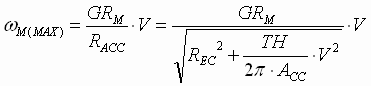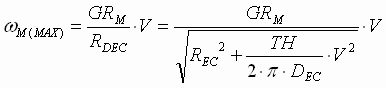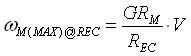; (16)

wM(MAX) is calculated with the minimum strip/web thickness, which results in minimum build-up in the roll during acceleration, and hence, maximum rotational velocity at RACC. A similar argument can be made for unwind applications.

For the system in Example 1.

wM(MAX) = 159 [rad/sec] or 1517 [rpm]

wM(MAX)@REC = 833 [rad/sec] or 7958 [rpm]

A significant difference. The observed difference is due to the use of a low acceleration rate and a small core radius. If a more reasonable acceleration rate is used, such as 50 [m/min/sec], we obtain:

wM(MAX) = 436 [rad/sec] or 4163 [rpm]

Still a significant difference. This shows that even with a 15:1 roll build-up ratio, a 1750 [rpm] base speed motor could be used with only a 2.3:1 field range. This shows clearly that a motor with a much smaller frame than the one resulting from a design using the theoretical maximum rotational speed, can be used in this application. The larger the core, and the thinner the material, the less this effect will be observed.

To take advantage of this observation in winding applications, an algorithm may be deployed that weakens the field based on rotational speed reference during acceleration, then switches to field strengthening as a function of Ri/RACC at radii greater than RACC. In unwinding applications the algorithm may weaken the field as a function of Ri/RDEC at radii greater than RDEC and then switch to field strengthening based on rotational speed reference during deceleration.

In some applications, the field may reach full strength at a radius less than RFR during run. This implies that the motor, which must be sized with adequate torque to maintain maximum application tension at full roll, may be significantly oversized at core, and be wasting significant amounts of horsepower for much of its operating time. It is always best to size the motor such that the radius at which the motor reaches full field strength is RFR, and adjust the acceleration(winder)/deceleration(unwinder) rates so that maximum fully-weakened-field motor speed is realized at RACC in winding applications, and RDEC in unwinding applications. If this methodology is employed in the application, and maximizing torque at core is not required, the field may be left fully weakened from core to RACC in winding applications, and from RDEC to core in unwinding applications.

A full description of the methodology behind selecting a motor and specifying a speed and field reference algorithm for a winding/unwinding application is outside the scope of this paper. It must take into consideration such phenomena as: 1) Motor over-torqueing duty cycles and the amount of over-torqueing that will be required of the motor while accelerating and decelerating the system inertia at full roll 2) Torque required to overcome system friction and windage losses 3) Tension tapering profiles that minimize starring, and telescoping phenomena during winding 4) Accumulator storage, and process line speeds 5) The relationship between process thruput, overhead costs and profit margins. It is left as the subject of discussion in a future paper.

In applications that lack a clean (digital) motor speed reference that is calculated based on a master line speed reference and measured or estimated roll radius, the equations that model rotational motor speed can be used to provide a feed-forward reference to the algorithm that controls field weakening. This helps to alleviate the poor performance that is often encountered in those applications where speed feedback is used as the reference for field weakening algorithms in Maxi-torque schemes. This is especially true when a closed loop trim algorithm designed to prevent the armature voltage from exceeding its rated value, is summed into the field reference.

If the system allows for scheduling of the acceleration and deceleration rates, Eq. (17) can be used to schedule these rates based on a given strip thickness, and a maximum rotational motor speed.

Winding/Unwinding [m/sec^2]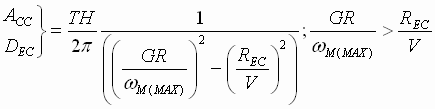(17)

IV. Conclusions

The set of equations presented in this paper provide a framework for scheduling winding and unwinding operations in strip/web processing lines. The potential application of these equations is broad in scope. They can be used to optimize many aspects of winding, including providing guidance in selecting motors. It is left up to the reader to identify specific applications in which they may be applied, based on a given set of operational requirements. The author has employed these and other equations found in , , , ,  in a well received proprietary optimization program that configures recipes for winding and unwinding operations, while specifying the least expensive motor for the given application, and the specified design constraints.

Caveats

The equations presented in this paper are not complete in so far as the effects of applying an "s-curve" to the speed reference are not considered. Also, the effects of air-entrainment, and radial pressure are ignored. Given that the metals industries do not usually employ significant "s-curved" speed references, and both air-entrainment and radial pressure phenomena are only slightly significant in foil applications, the equations presented here-in can be deployed in those applications with little or no error in the results.

One final caveat is that the accuracy of the equations is linearly dependant on the accuracy of the material thickness measurement. For example, if the thickness measurement is incorrect by 1 part in 100, the calculated radius will also be incorrect by the same amount at any instant in time.

References

 B. T. Boulter, "The Effect of Speed Loop Bandwidths on System Natural Frequencies in Multi-Zone Strip Processing Lines" , Transactions of the IEEE-IAS, pp. 126 - 134 Jan/Feb. 1999,

 B. T. Boulter, " Improving Bridle Low-Speed Regulation Using Cascaded Current Followers ", Transactions of the IEEE-IAS, pp. 1074-1082, Jul/Aug 2001,

 B. T. Boulter, " Applying Drive Performance Specifications to Systems Applications – Part I, Speed Performance ", Transactions of the IEEE-IAS, pp. 1082-1088, Jul/Aug 2001,

 B. T. Boulter, " Applying Drive Performance Specifications to Systems Applications – Part II, Torque/Current Regulation", Proceedings of the 36th IEEE IAS Conference, Chicago IL. Oct. 2001,

 B. T. Boulter, " Applying Drive Performance Specifications to Systems Applications – Part III, Position Regulation", Proceedings of the 37th IEEE IAS Conference, Pittsburgh PA. Oct. 2002,